CBSE Class 8CBSE
Share

Books Shortlist
Your shortlist is empty

# Question Bank Solutions for CBSE Class 8 - CBSE - Mathematics

Subjects
Chapters
Subjects
Popular subjects
Chapters
Mathematics
< prev 1 to 20 of 3178 next >

For each of the given solid, the two views are given. Match for each solid the
corresponding top and front views.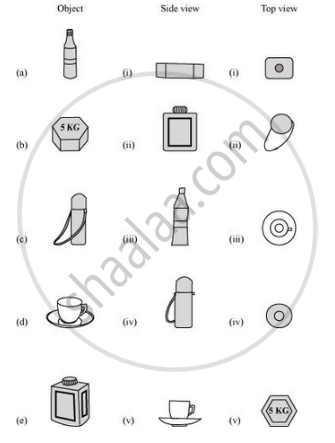Visualizing Solid Shapes
Chapter:  Visualizing Solid Shapes
Concept: Views of 3D-shapes

A square and a rectangular field with measurements as given in the figure have the same perimeter. Which field has a larger area?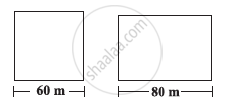Mensuration
Chapter:  Mensuration
Concept: Introduction of Mensuration

Mrs. Kaushik has a square plot with the measurement as shown in the following figure. She wants to construct a house in the middle of the plot. A garden is developed around the house. Find the total cost of developing a garden around the house at the rate of Rs 55 per m2.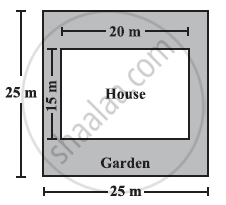Mensuration
Chapter:  Mensuration
Concept: Introduction of Mensuration

The shape of a garden is rectangular in the middle and semi circular at the ends as shown in the diagram. Find the area and the perimeter of the garden [Length of rectangle is 20 − (3.5 + 3.5) metres]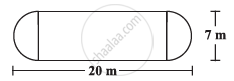Mensuration
Chapter:  Mensuration
Concept: Introduction of Mensuration

A flooring tile has the shape of a parallelogram whose base is 24 cm and the corresponding height is 10 cm. How many such tiles are required to cover a floor of area 1080 m2? (If required you can split the tiles in whatever way you want to fill up the corners).

 Mensuration
Chapter:  Mensuration
Concept: Introduction of Mensuration

An ant is moving around a few food pieces of different shapes scattered on the floor. For which food − piece would the ant have to take a longer round? Remember, circumference of a circle can be obtained by using the expression c = 2πr, where r is the radius of the circle.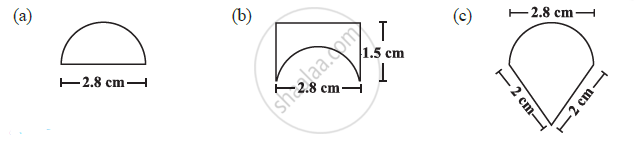Mensuration
Chapter:  Mensuration
Concept: Introduction of Mensuration

Evaluate 3−2

 Exponents and Powers
Chapter:  Exponents and Powers
Concept: Powers with Negative Exponents

Evaluate (−4)−2

 Exponents and Powers
Chapter:  Exponents and Powers
Concept: Powers with Negative Exponents

Evaluate (1/2)^(-5)

 Exponents and Powers
Chapter:  Exponents and Powers
Concept: Powers with Negative Exponents

Find the value of m for which 5m ÷5−3 = 55.

 Exponents and Powers
Chapter:  Exponents and Powers
Concept: Powers with Negative Exponents

Evaluate  {(1/3)^(-1) - (1/4)^(-1)}^(-1)

 Exponents and Powers
Chapter:  Exponents and Powers
Concept: Powers with Negative Exponents

Evaluate (5/8)^(-7) xx (8/5)^(-4)

 Exponents and Powers
Chapter:  Exponents and Powers
Concept: Powers with Negative Exponents

For the given solid, the three views are given. Identify for each solid the corresponding top, front and side views.

 Object (i) (ii) (iii)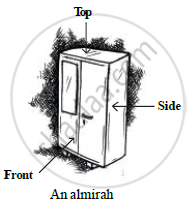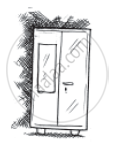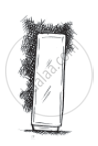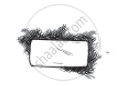Visualizing Solid Shapes
Chapter:  Visualizing Solid Shapes
Concept: Views of 3D-shapes

For the given solid, the three views are given. Identify for each solid the corresponding top, front and side views.

 Object (i) (ii) (iii)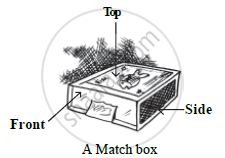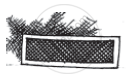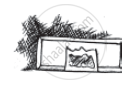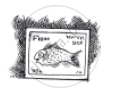Visualizing Solid Shapes
Chapter:  Visualizing Solid Shapes
Concept: Views of 3D-shapes

For the given solid, the three views are given. Identify for each solid the corresponding top, front and side views.

 Object (i) (ii) (iii)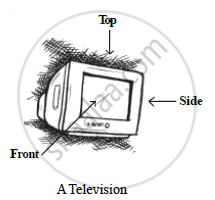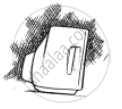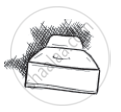Visualizing Solid Shapes
Chapter:  Visualizing Solid Shapes
Concept: Views of 3D-shapes

For the given solid, the three views are given. Identify for each solid the corresponding top, front and side views.

 Object (i) (ii) (iii)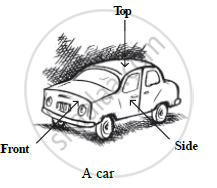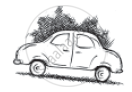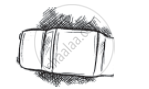Visualizing Solid Shapes
Chapter:  Visualizing Solid Shapes
Concept: Views of 3D-shapes

For the given solid, identify the top view, front view and side view.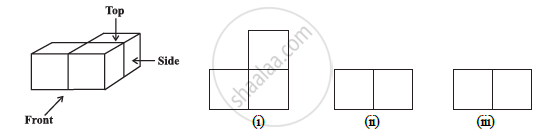Visualizing Solid Shapes
Chapter:  Visualizing Solid Shapes
Concept: Views of 3D-shapes

For the given solid, identify the top view, front view and side view.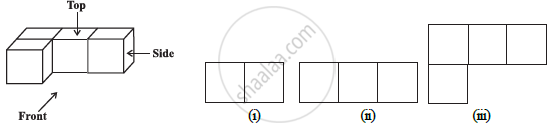Visualizing Solid Shapes
Chapter:  Visualizing Solid Shapes
Concept: Views of 3D-shapes

For the given solid, identify the top view, front view and side view.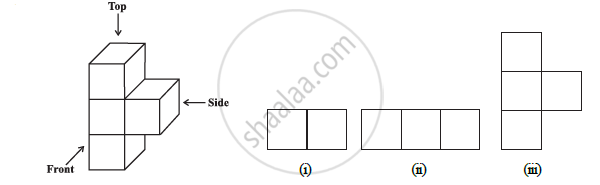Visualizing Solid Shapes
Chapter:  Visualizing Solid Shapes
Concept: Views of 3D-shapes

For the given solid, identify the top view, front view and side view.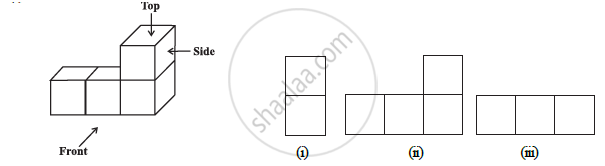Visualizing Solid Shapes
Chapter:  Visualizing Solid Shapes
Concept: Views of 3D-shapes
< prev 1 to 20 of 3178 next >
Question Bank Solutions for CBSE Class 8 CBSE Mathematics. You can further filter Question Bank Solutions by subjects and chapters. Solutions for most of the questions for CBSE can be found here on Shaalaa.com. You can use these solutions to prepare for your studies and ace in exams. Solving questions is a great way to practice and with Shaalaa.com, you can answer a question and then also check your answer with the solutions provided.
S